Updated date:

# How to divide an obtuse angle into 4 equal parts:

Author:

This hub answers ivs357's question on how to divide an obtuse angle into four equal parts. Firstly an obtuse angle is an angle which is greater than 90 degrees. This method could also be used to divide an acute angle into four equal parts. An acute angle is an angle less than 90 degrees.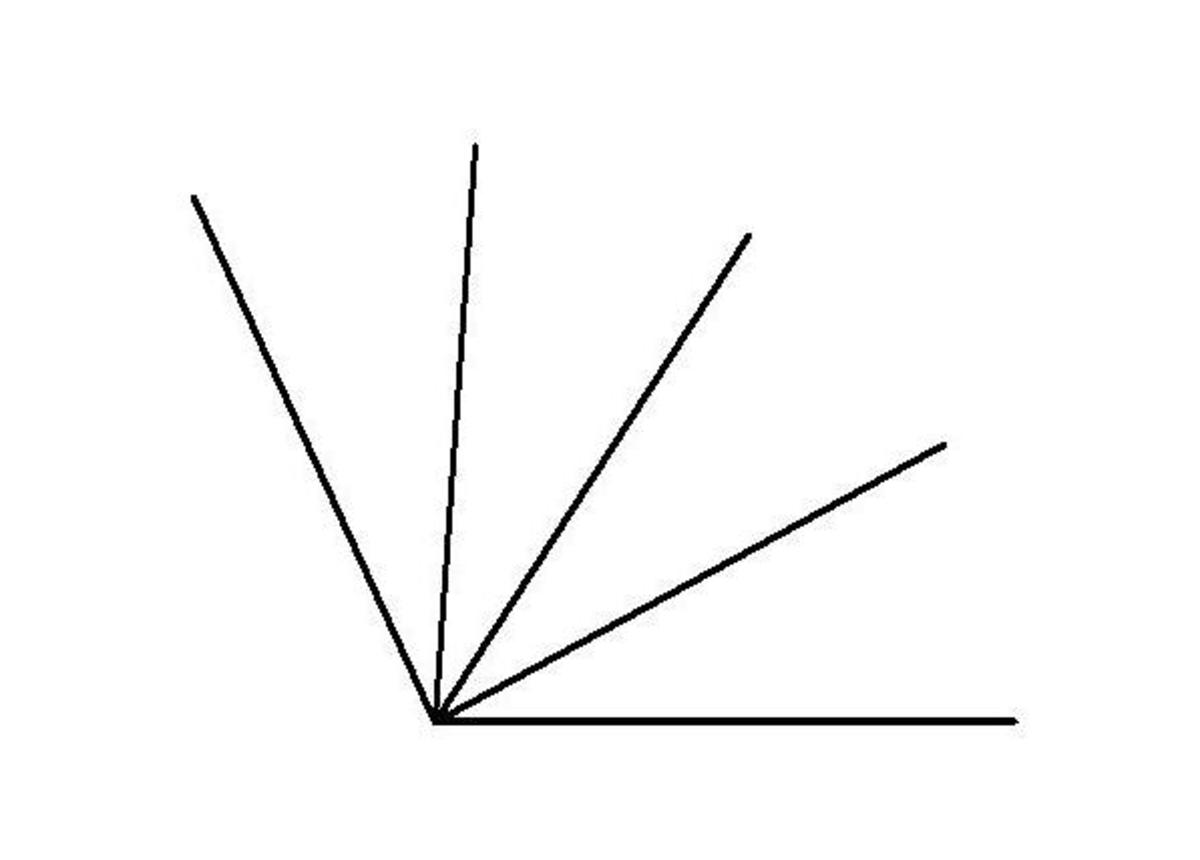The figure above shows an obtuse angle divided into 4 equal parts. From using this procedure you should be able to easily divide any angle into four equal parts.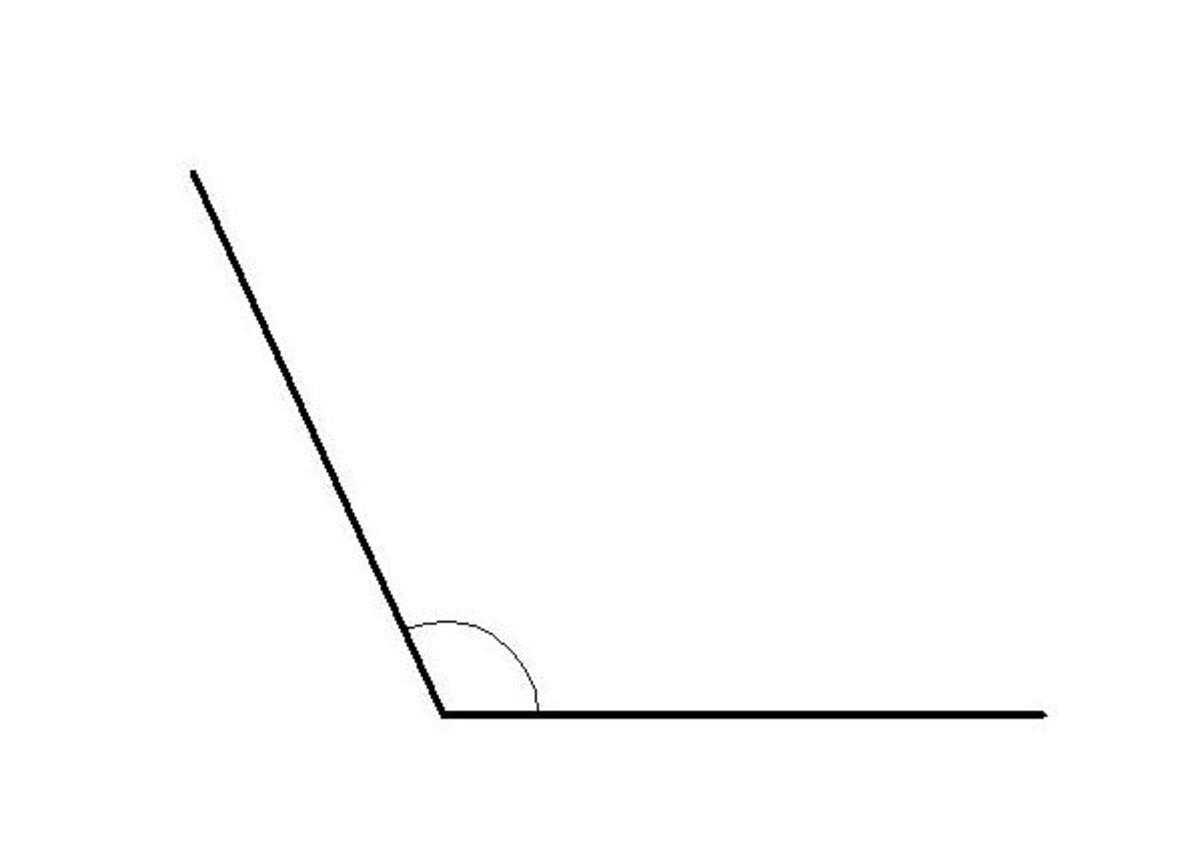Firstly lets start by drawing an obtuse angle, like the one shown in the above figure.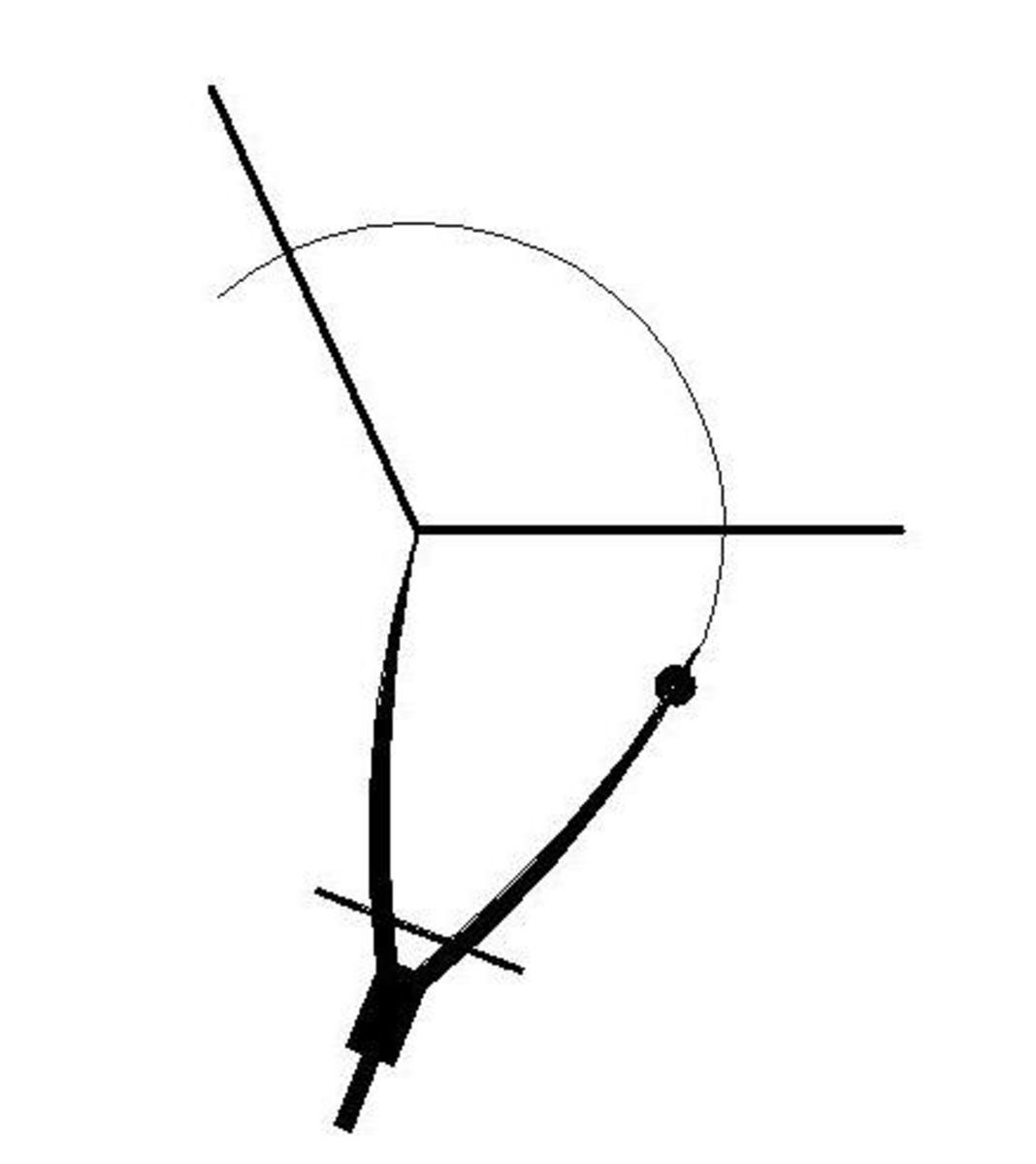Now with your compass, scribe an arc at any radius and bisect both sides of the obtuse angle as shown if the figure above.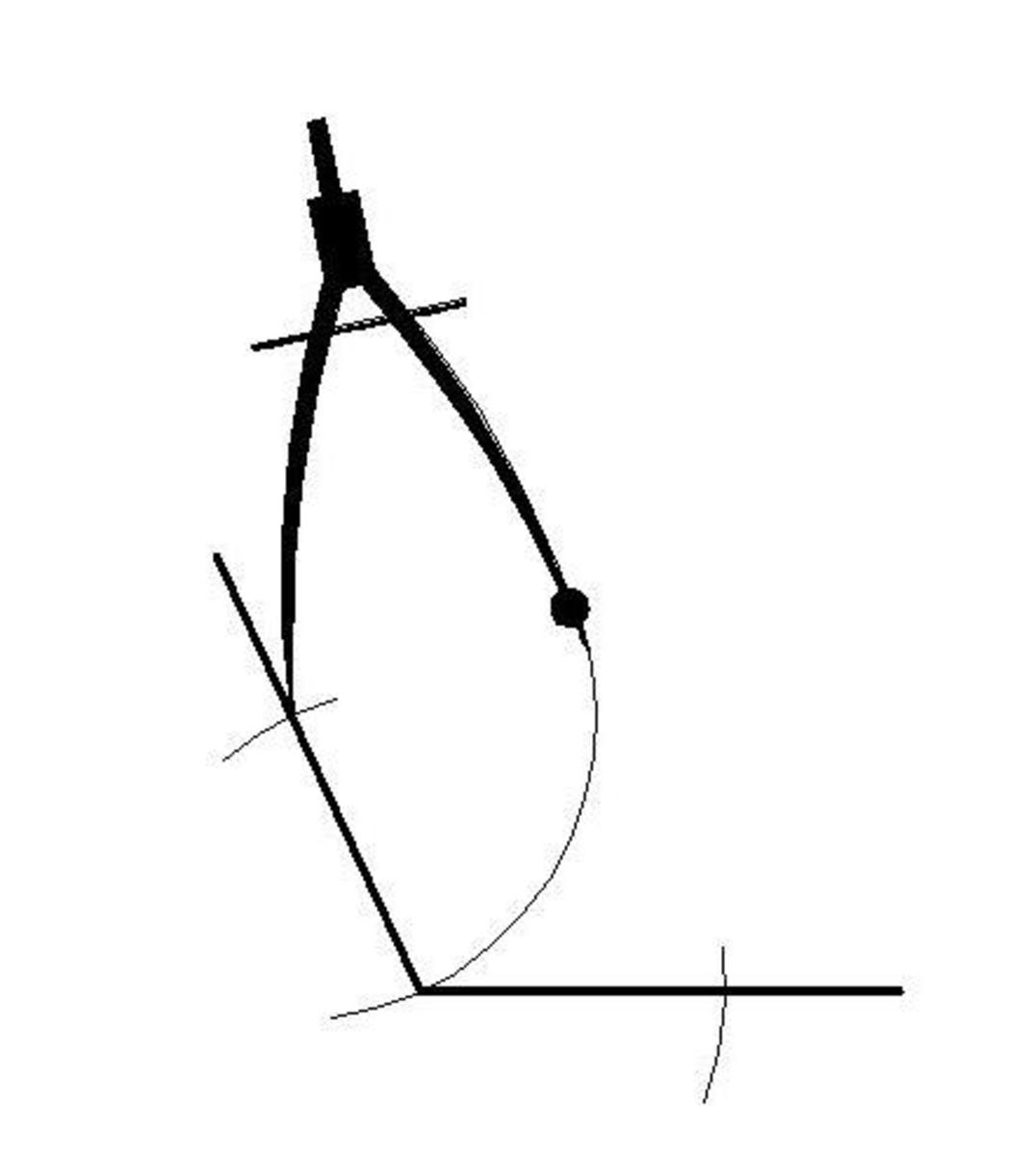With the same radius of your compass now scribe an arc from each of these intersections that you created with the previous step.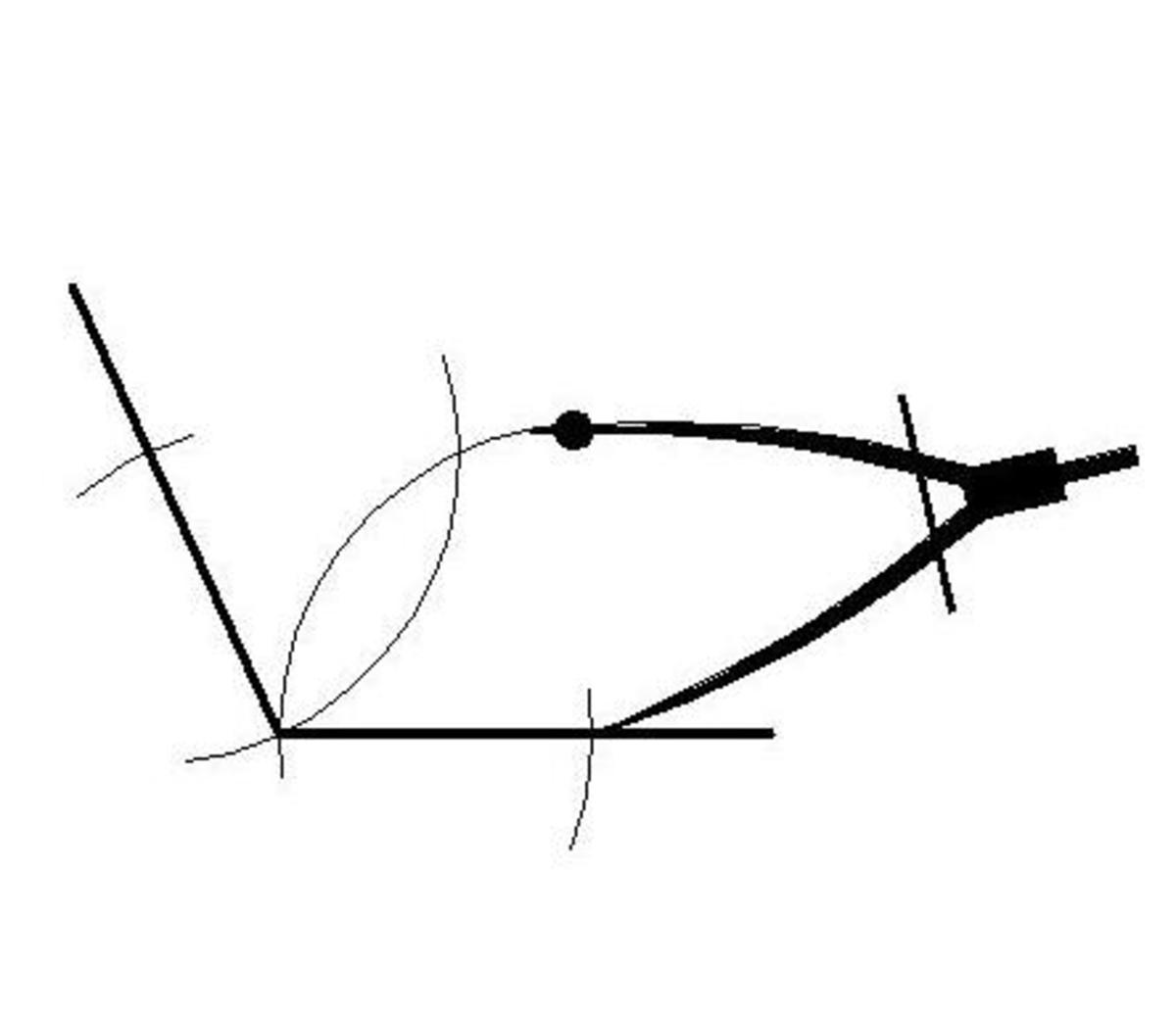You should intersect each of these arcs to form a cross. The intersection of these arcs for the point that you are after as shown in the figure above.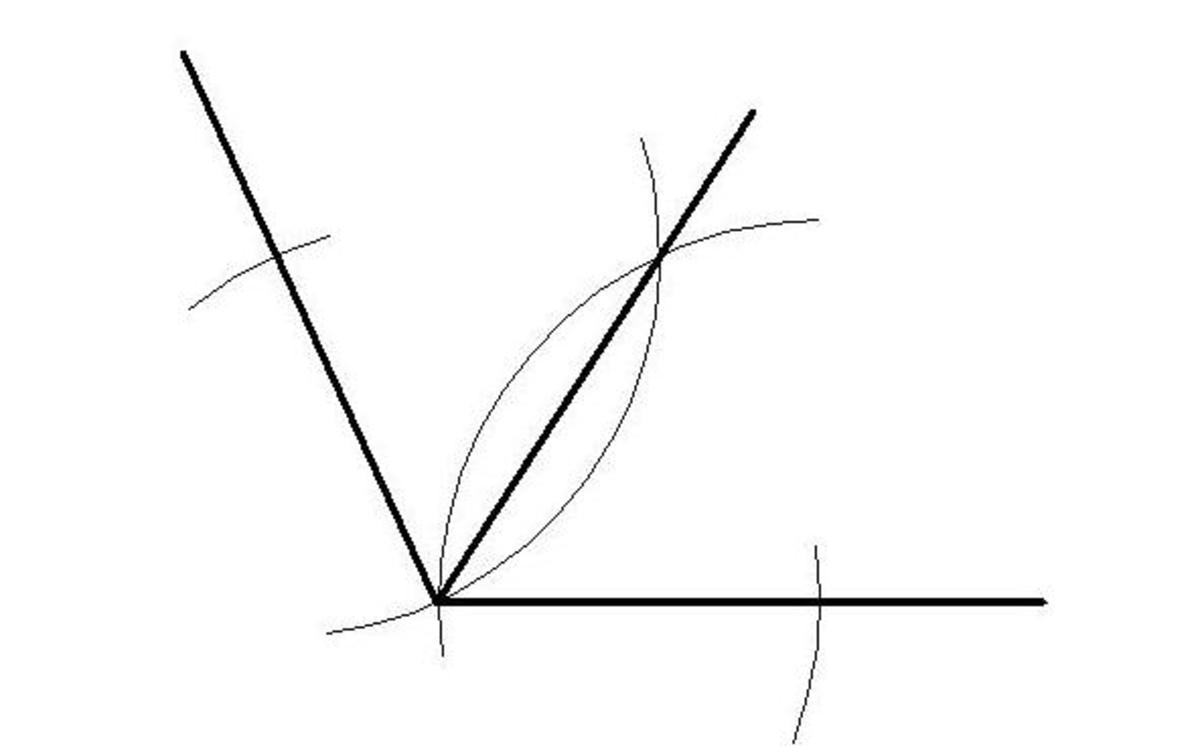Now draw a line from the start of your obtuse angle to the intersection of these arcs as shown in the above diagram.Now your obtuse angle should be divided into 2 equal parts as shown in the figure above. To divide your obtuse angle into 4 equal parts you simply repeat the above process.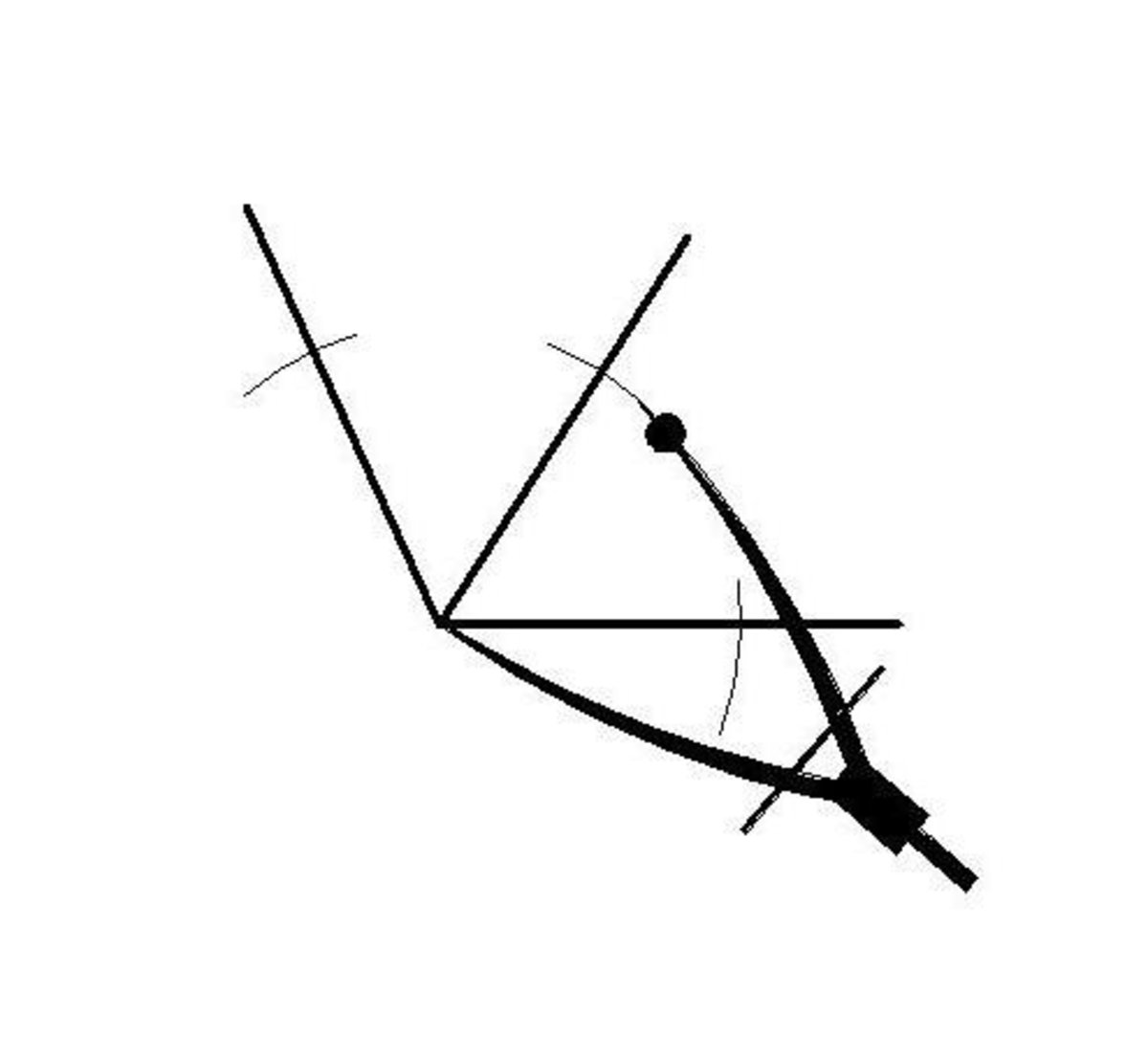With your compass scribe two arcs which bisect the sides of the lines which make up the newly divided angle as shown in the figure above.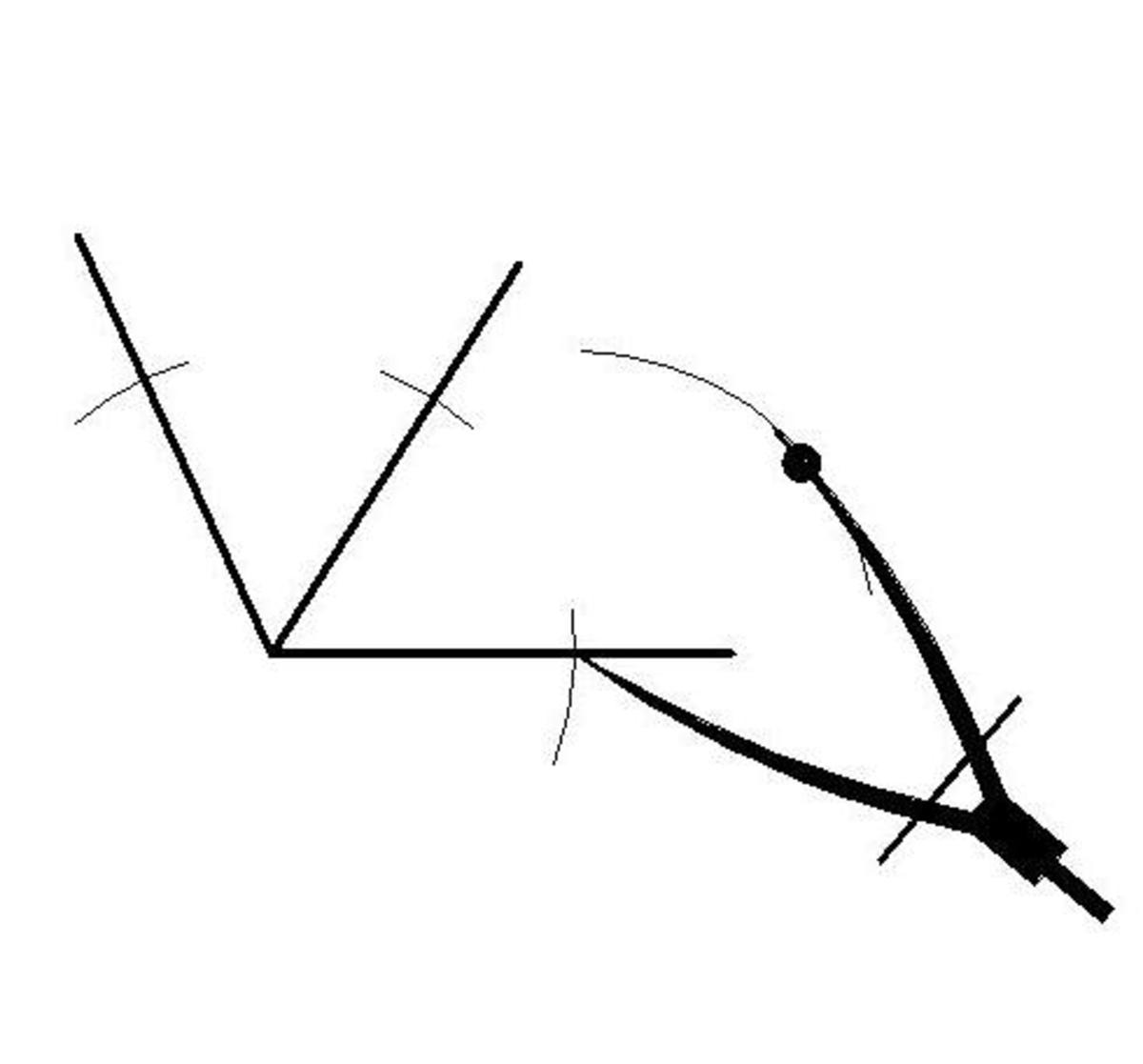With the same radius in your compass scribe two intersecting arcs from these bisection points (the small arcs which you just formed on the sides of the newly divide obtuse angle) as shown in the diagram above.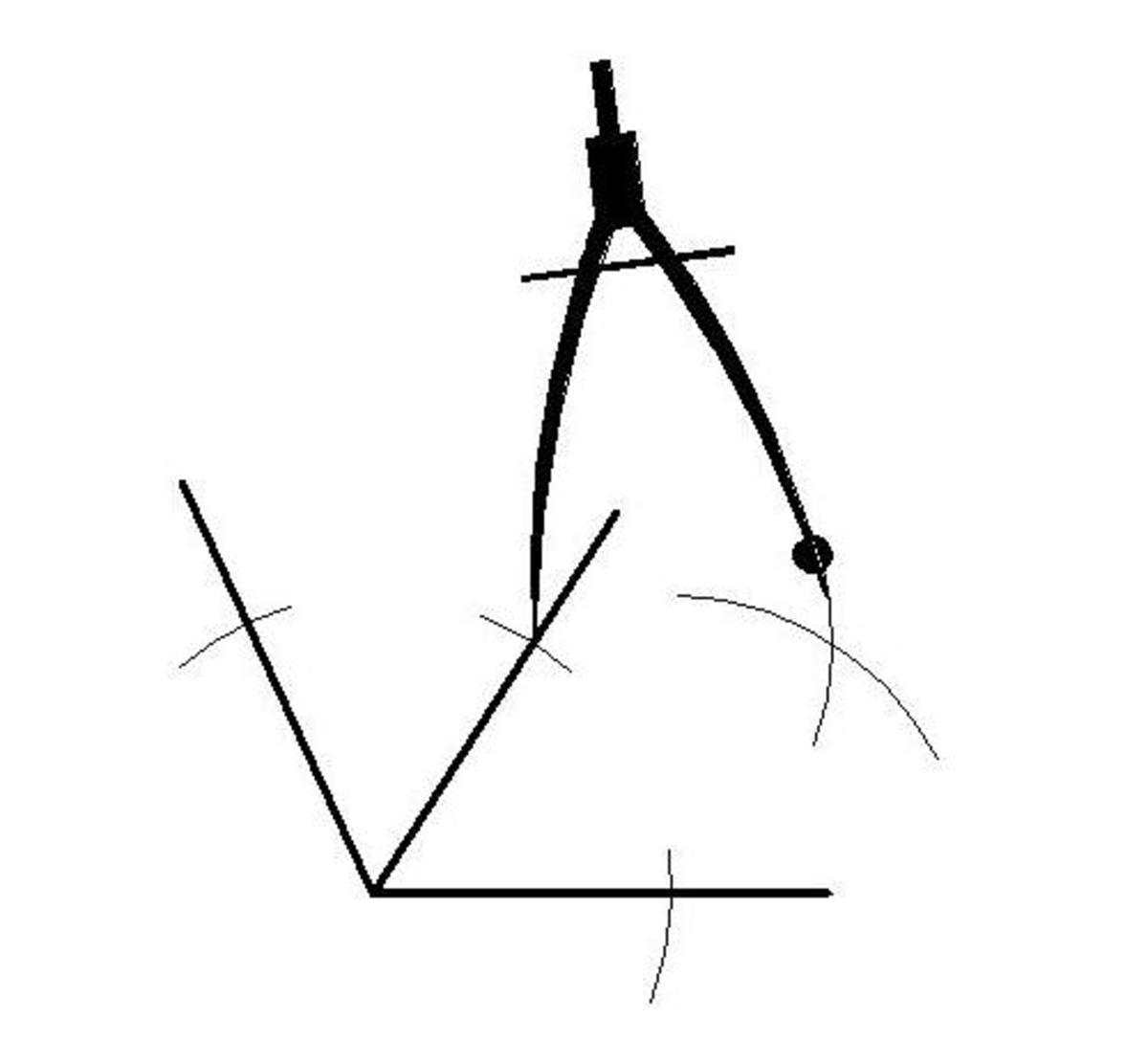The above diagram shows the completion of the intersecting arcs.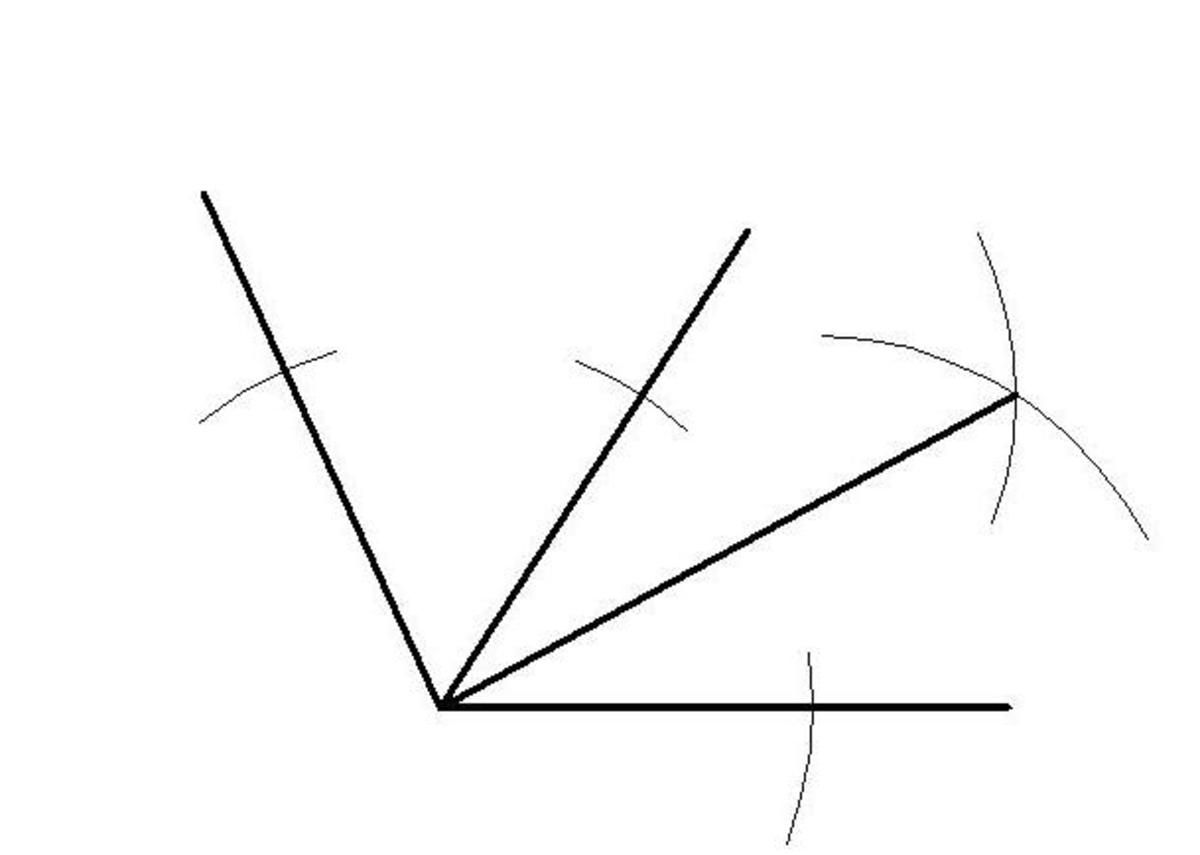Now draw a line from the base of the obtuse angle to the intersection of these arcs as shown in the diagram above.Repeat this process again for the other side of the obtuse angle and you will have your obtuse angle divided evenly up into 4 equal parts.

## Need some help with other geometry problems?

How to develop a cone.

How to develop a pyramid.

How to develop a cylinder.

How to draw fast in AutoCAD.

## Comments

LeonJane (author) from Australia on June 03, 2014:

Thanks Millionaire Tips for reading, hope it helps.